## 12.1: Three-Dimensional Coordinate Systems

### Distance Formula for 3-Dimensions

$$d = \sqrt{(x_2 - x_1)^2 + (y_2 - y_1)^2 + (z_2 - z_1)^2}$$
Derivation: Pythagorean Theorem

### Equation of a Sphere

Center, $C(h, k, l)$ and radius, $r$
$$(x-h)^2 + (y-k)^2 + (z-l)^2 = r^2$$

### Exercises

#### 12.1 Problem 9

Find the lengths of the side of the triangle $PQR$. Is it a right triangle? Is it an isosceles triangle?

$P(3, -2, -3), \ Q(7, 0, 1),\ R(1, 2, 1)$
$$s_1 = PQ = \sqrt{(x_p - x_q)^2 + (y_p - y_q)^2 + (z_p - z_q)^2} = \sqrt{4^2 + 2^2 + 4^2} = \sqrt{36} = 6$$
$$s_2 = QR = \sqrt{(x_q - x_r)^2 + (y_q - y_r)^2 + (z_q - z_r)^2} = \sqrt{6^2 + 2^2 + 0^2} = \sqrt{40} = 2\sqrt{10}$$
$$s_3 = PR = \sqrt{(x_p - x_r)^2 + (y_p - y_r)^2 + (z_p - z_r)^2} = \sqrt{2^2 + 4^2 + 4^2} = \sqrt{36} = 6$$
Isosceles Triangle
Not a right triangle since $6^2 + 6^2 \neq 40$ (sides are not a pythagorean triplet)

#### 12.1 Problem 13

Find an equation of the sphere with center (-3, 2, 5) and radius 4. What is the intersection of this sphere with the $yz$-plane?
$$(x-h)^2 + (y-k)^2 + (z-l)^2 = r^2$$
$$(x+3)^2 + (y-2)^2 + (z-5)^2 = 4^2$$
$$(x+3)^2 + (y-2)^2 + (z-5)^2 = 16$$

The intersection with the $yz$-plane will yield a circle.

Let $r_2$ be the radius of the circle created from the intersection.$$r_2 = \sqrt{4^2 - 3^2} = \sqrt{7}$$

Thus the equation of the intersecting circle is
$$(z - 5)^2 + (y-2)^2 = r_2^2$$
$$(z - 5)^2 + (y-2)^2 = 7$$

#### 12.1 Problem 15

Find an equation of the sphere that passes through the point (4, 3, -1) and has center (3, 8, 1)
$$r = d = \sqrt{(4-3)^2 + (3-8)^2 + (-1 - 1)^2} = \sqrt{1 + 25 + 4} = \sqrt{30}$$
$$r^2 = (x-3)^2 + (y-8)^2 + (z - 1)^2 = 30$$

## 12.2: Vectors

Vector: Quantity with both magnitude and direction (denoted v or $\vec{v}$ and graphically as an arrow)

• Initial Point indicated by the “tail” of the arrow and the ending point is represented by the “head” of the arrow

### Combining Vectors

• $\overrightarrow{AB} + \overrightarrow{BC} = \overrightarrow{AC}$

• The Triangle Law and Parallelogram Law are just ways of geometrically representing vector addition.

• Scalar Multiplication: If $c$ is a scalar and $v$ is a vector, then cv is just the vector, $v$ with length multiplied by $|c|$. If $v = 0$, then $cv = 0$

• Multiplying a vector by -1 means flipping the direction of the vector

• $u - v = u + (-v)$

### Components

• If a vector, $a$ starts at the origin, then the coordinates are either $(a_1, a_2)$ or $(a_1, a_2, a_3)$ (2d vs. 3d)
• These coordinates are called the components of $a$ and denoted with $\ev{a_1, a_2}$ or $\ev{a_1, a_2, a_3}$
Given the points $A(x_1, y_1, z_1)$ and $B(x_2, y_2, z_2)$, the vector $\textbf{a}$ with representation $\overrightarrow{AB}$ is
$$\textbf{a} = \ev{x_2 - x_1, y_2 - y_1, z_2 - z_1}$$
• The magnitude/length of the vector $\textbf{v}$ is the following:
$$|\textbf{a}| = \sqrt{a_1^2 + a_2^2 + a_3^2}$$

### Properties of Vectors

1. $\textbf{a + b = b + a}$
2. $\textbf{a + 0 = a}$
3. $c(\textbf{a + b}) = c\textbf{a} + c\textbf{b}$
4. $(cd)\textbf{a} = c(d\textbf{a})$
5. $\textbf{a + (b + c) = (a + b) + c}$
6. $\textbf{a + (-a) = 0}$
7. $(c + d)\textbf{a} = c\textbf{a} + d\textbf{a}$
8. $1\textbf{a = a}$

### Standard Basis Vectors and Unit Vectors

$$\textbf{i} = \ev{1, 0, 0}$$
$$\textbf{j} = \ev{0, 1, 0}$$
$$\textbf{k} = \ev{0, 0, 1}$$
$\textbf{i}$, $\textbf{j}$, and $\textbf{k}$ are Standard Basis Vectors which point in the direction of the x, y, or z axis and have a length of 1

• If a = $\ev{a_1, a_2, a_3}$, then a = $a_1 \textbf{i} + a_2 \textbf{j} + a_3 \textbf{k}$

Unit Vector: Vector with length 1 (So i, j, and k are examples of unit vectors)

• If $a \neq 0$, then the unit vector with the same direction as a is
$$\textbf{u} = \frac{\textbf{a}}{|\textbf{a}|}$$

### Applications

• Acceleration and Velocity
• Force
• The resultant force or net force can be found by vector addition of the individual forces acting on an object

### Exercises

#### Problem 2

What is the relationship between the point (4, 7) and the vector $\ev{4, 7}$? Illustrate with a sketch.

If the vector’s tail or starting point is located at the origin, then the head will be at the point (4, 7).#### Problem 3

Name all the equal vectors in the parallelogram shown.$$\overrightarrow{EA} = \overrightarrow{CE}\ \ \ \ \ \ \ \ \overrightarrow{DE} = \overrightarrow{EB}\ \ \ \ \ \ \ \ \overrightarrow{DA} = \overrightarrow{CB} \ \ \ \ \ \ \ \overrightarrow{AB} = \overrightarrow{DC}$$

#### Problem 4

Write each combination of vectors as a single vector.a. $\overrightarrow{AC}$
b. $\overrightarrow{CB}$
c. $\overrightarrow{DB} - \overrightarrow{AB} = \overrightarrow{DB} + \overrightarrow{BA} = \overrightarrow{DA}$
d. $\overrightarrow{DC} + \overrightarrow{CA} + \overrightarrow{AB} = \overrightarrow{DA} + \overrightarrow{AB} = \overrightarrow{DB}$

#### Problem 6

Copy the vectors in the figure and use them to draw the following vectors.#### Problem 7

In the figure, the tip of c and the tail of d are both the midpoint of $QR$. Express c and d in terms of a and b.$$c + d = b$$
$$c + (-d) = a$$

$$(c+d) + (c - d) = b+a$$
$$2c = b + a \implies c = \frac{b + a}{2}$$

$$\frac{b+a}{2} + d = b$$
$$d = b - \frac{b + a}{2} = \frac{2b - (b + a)}{2} = \frac{b - a}{2}$$

#### Problem 8

If the vectors in the figure satisfy |u| = |v| = 1 and u + v + w = 0, what is |w|?|w| = $\sqrt{2}$

#### Problem 9

Find a vector a with representation given by the directed line segment $\overrightarrow{AB}$. Draw $\overrightarrow{AB}$ and the equivalent representation starting at the origin.
$$A(-2, 1), \ \ B(1, 2)$$
$$\textbf{a} = \ev{1 - (-2), 2 - 1} = \ev{3, 1}$$#### Problem 13

Find a vector a with representation given by the directed line segment $\overrightarrow{AB}$. Draw $\overrightarrow{AB}$ and the equivalent representation starting at the origin.
$$A(0, 3, 1), \ \ B(2, 3, -1)$$
$$\textbf{a} = \ev{2 - 0, 3 - 3, -1 - 1} = \ev{2, 0, -2}$$

#### Problem 15

Find the sum of the given vectors and illustrate geometrically.

$$\ev{-1, 4}, \ \ \ev{6, -2}$$
$$\ev{6 + (-1), -2 + 4} = \ev{5, 2}$$

#### Problem 17

Find the sum of the given vectors and illustrate geometrically.
$$\ev{3, 0, 1}, \ \ \ev{0, 8, 0}$$
$$\ev{3 + 0, 0 + 8, 1 + 0} = \ev{3, 8, 1}$$

#### Problem 19

Find a + b, 4a + 2b, |a|, and |a - b|.
a = $\ev{-3, 4}$, b = $\ev{9, -1}$

a + b =
$$\ev{-3 + 9, 4 + (-1)} = \ev{6, 3}$$

4a + 2b =
$$(4) \ev{-3, 4} + (2) \ev{9, -1}$$
$$= \ev{-12, 16} + \ev{18, -2}= \ev{-12 + 18, 16 + (-2)}$$
$$= \ev{6, 14}$$

|a| =
$$\sqrt{(-3)^2 + 4^2} = \sqrt{9 + 16} = \sqrt{25} = 5$$

a - b =
$$\ev{-3, 4} - \ev{9, -1} = \ev{-3, 4} + \ev{-9, 1}$$
$$\ev{-3 + -9, 4 + 1} = \ev{-12, 5}$$

|a - b| =
$$\sqrt{(-12)^2 + 5^2} = \sqrt{144 + 25} = \sqrt{169} = 13$$

#### Problem 21

Find a + b, 4a + 2b, |a|, and |a - b|.
a = $4\textbf{i} - 3\textbf{j} + 2\textbf{k}$, b = $2\textbf{i} - 4\textbf{k}$

a + b =
$$(4 + 2)\textbf{i} + (-3 + 0)\textbf{j} + (2+ (-4))\textbf{k} = 6\textbf{i} -3\textbf{j} - 2\textbf{k}$$

4a + 2b =
$$4(4\textbf{i} - 3\textbf{j} + 2\textbf{k}) + 2(2\textbf{i} - 4\textbf{k}) = (16\textbf{i} - 12\textbf{j} + 8\textbf{k}) + (4\textbf{i} - 8\textbf{k}) = 20\textbf{i} -12\textbf{j} + 0\textbf{k}$$
$$= 20\textbf{i} - 12\textbf{j}$$

|a| =
$$\sqrt{4^2 + (-3)^2 + (2)^2} = \sqrt{16 + 9 + 4} = \sqrt{29}$$

a - b =

$$(4 - 2)\textbf{i} + (-3 - 0)\textbf{j} + (2 - (-4))\textbf{k} = 2\textbf{i} -3\textbf{j} + 6\textbf{k}$$

|a - b| =
$$\sqrt{2^2 + (-3)^2 + 6^2} = \sqrt{4 + 9 + 36} = \sqrt{49} = 7$$

#### Problem 23

Find a unit vector that has the same direction as the given vector.
$$\ev{6, -2}$$
$$\textbf{u} = \frac{\textbf{a}}{|\textbf{a}|} = \ev{6, -2} \frac{1}{\sqrt{6^2 + (-2)^2}} = \ev{6, -2} \frac{1}{\sqrt{40} } = \ev{6, -2} \frac{1}{2\sqrt{10}}$$
$$= \ev{\frac{6}{2\sqrt{10}}, \frac{-2}{2\sqrt{10} }} = \ev{\frac{3}{\sqrt{10}}, -\frac{1}{\sqrt{10}}}$$

#### Problem 25

Find a unit vector that has the same direction as the given vector.
$$8\textbf{i} - \textbf{j} + 4\textbf{k}$$
$$\textbf{u} = \frac{\textbf{a}}{|\textbf{a}|} = (8\textbf{i} - \textbf{j} + 4\textbf{k} ) \frac{1}{\sqrt{8^2 + (-1)^2 + 4^2}} = (8\textbf{i} - \textbf{j} + 4\textbf{k} ) \frac{1}{\sqrt{64 + 1 + 16} } =(8\textbf{i} - \textbf{j} + 4\textbf{k} ) \frac{1}{\sqrt{81} }$$
$$= (8\textbf{i} - \textbf{j} + 4\textbf{k} ) \frac{1}{9} = \frac{8}{9}\textbf{i} - \frac{1}{9}\textbf{j} + \frac{4}{9}\textbf{k}$$

#### Problem 26

Find the vector that has the same direction as $\ev{6, 2, -3}$ but has length 4.

$$\textbf{u} = \frac{\textbf{a}}{|\textbf{a}|} = \ev{6, 2, -3} \frac{1}{\sqrt{6^2 + 2^2 + (-3)^2}} = \ev{6, 2, -3} \frac{1}{36 + 4 + 9} = \ev{6, 2, -3} \frac{1}{\sqrt{49}} = \ev{\frac{6}{7}, \frac{2}{7}, -\frac{3}{7}}$$
$$4\textbf{u} = 4 \ev{\frac{6}{7}, \frac{2}{7}, -\frac{3}{7}} = \ev{\frac{24}{7}, \frac{8}{7}, -\frac{12}{7}}$$

#### Problem 29

If v lies in the first quadrant and makes an angle $\frac{\pi}{3}$ with the positive $x$-axis and |v| = 4, find v in component form.$$\ev{4\cos{\frac{\pi}{3}}, 4\sin{\frac{\pi}{3}}} = \ev{\frac{4(1)}{2}, \frac{4 \sqrt{3} }{2}}$$
$$\ev{2, 2\sqrt{3}}$$

#### Problem 43

If $A$, $B$, and $C$ are the vertices of a rectangle, find $\overrightarrow{AB} + \overrightarrow{BC} + \overrightarrow{CA}$

$$\overrightarrow{AB} + \overrightarrow{BC} + \overrightarrow{CA} = \overrightarrow{AC} + \overrightarrow{CA} = \overrightarrow{AA} = 0$$

#### Problem 44

Let $C$ be the point on the line segment $AB$ that is twice as far from $B$ as it is from $A$. If $\textbf{a} = \overrightarrow{OA}$, $\textbf{b} = \overrightarrow{OB}$, and $\textbf{c} = \overrightarrow{OC}$, show that $\textbf{c} = \frac{2}{3} \textbf{a} + \frac{1}{3}\textbf{b}$.$$-\textbf{a} = \overrightarrow{AO}$$
$$-a + b = \overrightarrow{AO} + \overrightarrow{OB} = \overrightarrow{AB}$$
$$\overrightarrow{AB} = \overrightarrow{AC} + \overrightarrow{CB} = \frac{1}{2}\overrightarrow{CB} + \overrightarrow{CB}$$

$$\overrightarrow{CO} + \overrightarrow{OB} = \overrightarrow{CB} = -\textbf{c} + \textbf{b}$$

$$\overrightarrow{AB} = \frac{1}{2}\overrightarrow{CB} + \overrightarrow{CB} = \frac{1}{2}(-c + b) + (-c + b)$$

$$(-a + b) = \frac{3}{2}(b - c)$$
$$\frac{2}{3}b - \frac{2}{3}a = b - c$$

$$\frac{2}{3}b - b - \frac{2}{3}a = -c$$
$$b - \frac{2}{3}b + \frac{2}{3}a = c$$
$$c = \frac{1}{3}b + \frac{2}{3}a$$

#### Problem 45

a. Draw the vectors a = $\ev{3, 2}$, b = $\ev{2, -1}$, and c = $\ev{7, 1}$.

b. Show, by means of a sketch, that there are scalars $s$ and $t$ such that $\textbf{c} = s\textbf{a} + t\textbf{b}$.#### Problem 47

If $\textbf{r} = \ev{x, y, z}$ and $\vb{r_0} = \ev{x_0, y_0, z_0}$, describe the set of all points $(x, y, z)$ such that $|\vb{r} - \vb{r_0}| = 1$.

$$\vb{r} - \vb{r_0} = \ev{x - x_0, y-y_0, z-z_0}$$
$$|\vb{r} - \vb{r_0}| = \sqrt{(x-x_0)^2 + (y-y_0)^2 + (z-z_0)^2} = 1$$
$$(x-x_0)^2 + (y-y_0)^2 + (z-z_0)^2 = 1^2$$
The set of all points will form a sphere with center $(x_0, y_0, z_0)$ with a radius of $1$.

## 12.3: The Dot Product

If $\vb{a} = \ev{a_1, a_2, a_3}$ and $\vb{b} = \ev{b_1, b_2, b_3}$, then the dot product of $\vb{a}$ and $\vb{b}$ is denoted by $\vb{a} \cdot \vb{b}$ and is calculated by the following
$$\vb{a} \cdot \vb{b} = a_1 b_1 + a_2 b_2 + a_3 b_3$$

The result of the dot product is a scalar quantity, not a vector.

### Properties of the Dot Product

If $\vb{a}, \vb{b}, \vb{c}$ are vectors in $V_3$ and $c$ is a scalar, then

1. $\vb{a} \cdot \vb{a} = |\vb{a}|^2$
2. $\vb{a} \cdot \vb{b} = \vb{b} \cdot \vb{a}$
3. $\vb{a} \cdot (\vb{b} + \vb{c}) = \vb{a} \cdot \vb{b} + \vb{a} \cdot \vb{c}$
4. $(c\vb{a}) \cdot \vb{b} = c (\vb{a} \cdot \vb{b}) = \vb{a} \cdot (c\vb{b})$
5. $0 \cdot \vb{a} = 0$

If $\theta$ is the angle between the vectors $\vb{a}$ and $\vb{b}$, then
$$\vb{a} \cdot \vb{b} = |\vb{a}||\vb{b}|\cos\theta$$

Two vectors $\vb{a}$ and $\vb{b}$ are perpendicular/orthogonal iff $\vb{a} \cdot \vb{b} = 0$

### Direction Angles and Direction Cosines

Direction Angles are the angles $\alpha, \beta, \gamma$ that $\vb{a}$ makes with the positive x-, y-, and z-axes, respectively.
$$\vb{a} = |\vb{a}|\ev{\cos\alpha, \cos \beta, \cos\gamma}$$

Unit vector in direction of $\vb{a}$:
$$u = \frac{1}{|\vb{a}|} \vb{a} = \ev{\cos \alpha, \cos \beta, \cos \gamma}$$

### Projections

• Vector Projection of $\vb{b}$ onto $\vb{a}$ is like the shadow of $\vb{b}$ in the direction of $\vb{a}$
• The scalar projection of $\vb{b}$ onto $\vb{a}$ (also called the component of b along a) is defined to be the signed magnitude of the vector projection
$$comp_a \vb{b} = \frac{\vb{a} \cdot \vb{b}}{|\vb{a}|} = |\vb{b}| \cos \theta$$
$$proj_a \vb{b} = \left(\frac{\vb{a} \cdot \vb{b}}{|\vb{a}|}\right) \frac{\vb{a}}{|\vb{a}|} = \frac{\vb{a} \cdot \vb{b}}{|\vb{a}|^2} \vb{a}$$

$\theta$ is just the angle between the vectors.
The vector projection is the scalar projection times the unit vector in the direction of $\vb{a}$

### Work

$W = \vb{F} \cdot \vb{d}$

## 12.4: The Cross Product

• The Cross Product of $\vb{a}$ and $\vb{b}$ gives a vector that is perpendicular to both vectors (use right hand rule to find out direction of resultant vector)
• If $\vb{a} = \ev{a_1, a_2, a_3}$ and $\vb{b} = \ev{b_1, b_2, b_3}$, then the cross product of $\vb{a}$ and $\vb{b}$ is the vector
$$\vb{a} \times \vb{b} = \ev{a_2b_3 - a_3 b_2, a_3 b_1 - a_1b_3, a_1b_2, a_1b_2 - a_2 b_1}$$
Derivation: define a vector $\vb{c}$ that is perpendicular to both $\vb{a}$ and $\vb{b}$ such that the dot product is 0
• Matrices can represent the above in a simpler fashion and less arbitrary looking way:

$$\vb{a} \cross \vb{b} = \begin{bmatrix} \vb{i} & \vb{j} & \vb{k} \\ a_1 & a_2 & a_3 \\ b_1 & b_2 & b_3 \end{bmatrix} = \begin{bmatrix} a_2 & a_3 \\ b_2 & b_3 \end{bmatrix} \vb{i} - \begin{bmatrix} a_1 & a_3 \\ b_1 & b_3 \end{bmatrix} \vb{j} + \begin{bmatrix} a_1 & a_2 \\ b_1 & b_2 \end{bmatrix} \vb{k}$$

• Notice how the terms each have a unit vector and the determinant order 2 matrix is constructed from the original order 3 matrix with the row and column of the unit vector removed.

• The determinant of order 2:
$$\begin{bmatrix} a & b \\ c & d \end{bmatrix} = ad - bc$$

• If $\theta$ is the angle between $\vb{a}$ and $\vb{b}$, then $|\vb{a} \cross \vb{b}| = |\vb{a} \mid \vb{b}| \sin\theta$

• Two nonzero vectors $\vb{a}$ and $\vb{b}$ are parallel if and only if $\vb{a} \cross \vb{b} = 0$

• The length of the cross product is equal to the area of the parallelogram formed by $\vb{a}$ and $\vb{b}$

• The cross product is NOT commutative or associative

### Properties of the Cross Product

1. $\vb{a} \cross \vb{b} = -\vb{b} \cross \vb{a}$
2. $(c\vb{a})\cross \vb{b} = c(\vb{a} \cross \vb{a}) = \vb{a} \cross (c\vb{b})$
3. $\vb{a} \cross (\vb{b} + \vb{c}) = \vb{a} \cross \vb{b} + \vb{a} \cross \vb{c}$
4. $(\vb{a} + \vb{b}) \cross \vb{c} = \vb{a} \cross \vb{c} + \vb{b} \cross \vb{c}$
5. $\vb{a} \cdot (\vb{b} \cross \vb{c}) = (\vb{a} \cross \vb{b}) \cdot \vb{c}$
6. $\vb{a} \cross (\vb{b} \cross \vb{c}) = (\vb{a} \cdot \vb{c}) \vb{b} - (\vb{a} \cdot \vb{b}) \vb{c}$

### Triple Products

• Scalar triple product (Property 5):
$$\vb{a} \cdot (\vb{b} \cross \vb{c}) = \begin{bmatrix} a_1 & a_2 & a_3 \\ b_1 & b_2 & b_3 \\ c_1 & c_2 & c_3 \end{bmatrix}$$
• The scalar triple product describes the volume of the parallelepiped
• Vector triple product: Property 6

### Torque

$|\vb{\tau}| = |\vb{r} \cross \vb{F}|$

## 12.5: Equations of Lines and Planes

### Lines

$L$ is a line in 3d space. The vector equation of $L$ is the following
$$\vb{r} = \vb{r_0} + t\vb{v}$$
where $t$ is a parameter, $\vb{r_0}$ is a position vector to a point on L, and $\vb{r}$ gives a point on L.

If $\vb{r} = \ev{x, y, z}$ and $\vb{r_0} = \ev{x_0, y_0, z_0}$,
the parametric equations for a line through the point ($x_0, y_0, z_0$) and parallel to the direction vector $\ev{a, b, c}$ are
$$x = x_0 + at, \ \ \ y = y_0 + bt, \ \ \ z = z_0 + ct$$

Symmetric Equations of L:
$$\frac{x-x_0}{a} = \frac{y-y_0}{b} = \frac{z-z_0}{c}$$
If $a$, $b$, or $c$ are 0:

• Example: If $a = 0$, then $x_0 = x \ \ \ \ \frac{y-y_0}{b} = \frac{z-z_0}{c}$, and the line L lies in the vertical plane $x=x_0$

Line segment from $r_0$ to $r_1$:
$$\vb{r}(t) = (1-t)\vb{r_0} + t\vb{r_1} \ \ \ \ 0 \le t \le 1$$

### Planes

Vector Equation of the Plane:
$$\vb{n} \cdot (\vb{r} - \vb{r_0}) = 0$$
$$\vb{n} \cdot \vb{r} = \vb{n} \cdot \vb{r_0}$$
$n$ is the normal vector (perpendicular to the plane)
$r_0$ and $r$ are position vectors
$r - r_0$ represent a line on the plane.

Scalar Equation of the Plane:
$$a(x-x_0) + b(y-y_0) + c(z - z_0) = 0$$
where $\vb{n} = \ev{a, b, c}$ and point $P_0(x_0, y_0, z_0)$ is on the plane

Linear Equation Form of the Scalar Equation of a Plane
$$ax + by + cz + d = 0$$
where $d = -(ax_0 + by_0 + cz_0)$

Two planes are parallel if their normal vectors are parallel.

### Distances

$$D = \frac{|ax_1 + by_1 + cz_1 + d|}{\sqrt{a^2 + b^2 + c^2}}$$
Derivation: The distance from an arbitrary point to a plane is the scalar projection of the distance between any point on the plane and the arbitrary point onto the normal vector.

## 12.6: Cylinders and Quadratic Surfaces

• Traces/Cross Sections: Curves of intersections of surfaces with planes parallel to the coordinate planes

• Steps for sketching surface:

• Find intercepts by settings two variables to zero and solving for third
• Make the “different” variable the axis that the surface is formed around (vertical axis) (e.g. all other variables on one side of equation or only one variable has negative)
• Determine the “traces” by holding one variable constant and seeing what the equation with two variables represents (hyperbola, parabola, or ellipse)

### Cylinders

• Cylinder: Surface with all lines (rulings) parallel to a given line and pass through a given plane curve.

• Equation only has two variables (e.g. $x^2 + y^2 = 1$, $y^2 + z^2 = 1$

• Example: $z = x^2$

• Let $z$ be the vertical axis

• If $y$ is set to be a constant, it doesn’t affect the equation and the traces formed by intersection with the xz plane will form parabolic cross sections since $z= x^2$ is a parabola when graphed on the x-z plane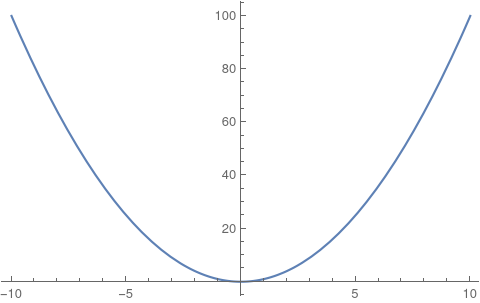• If $z$ is held constant, you have $c = x^2$, where c is a constant. This equation is just two lines on the xy plane. So the traces formed from the intersection with the xz plane will form cross sections that consist of two lines.

• If $x$ is held constant, you have $z = c$, where c is a constant. This is just the equation of one line on the yz plane.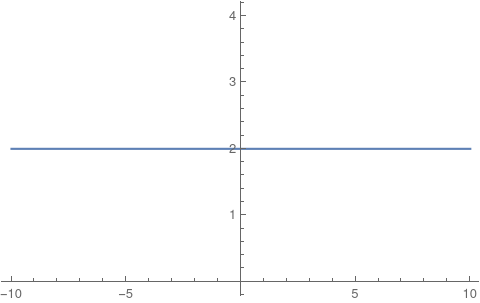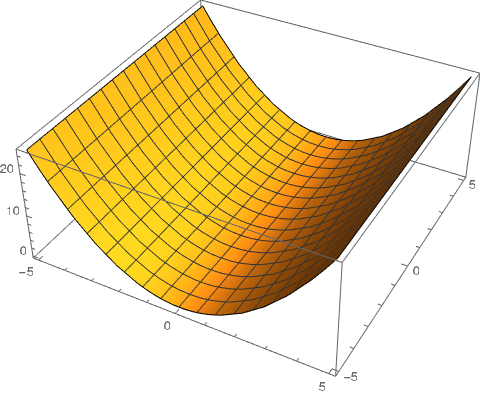• Equations have the form $Ax^2 + By^2 + Cz^2 + Dxy + Eyz + Fxz + Gx + Hy + Iz + J = 0$

#### Ellipsoid

• All traces are ellipses
• If $a = b= c$, the ellipsoid is a sphere
• $\frac{x^2}{a^2} + \frac{y^2}{b^2} + \frac{z^2}{c^2} = 1$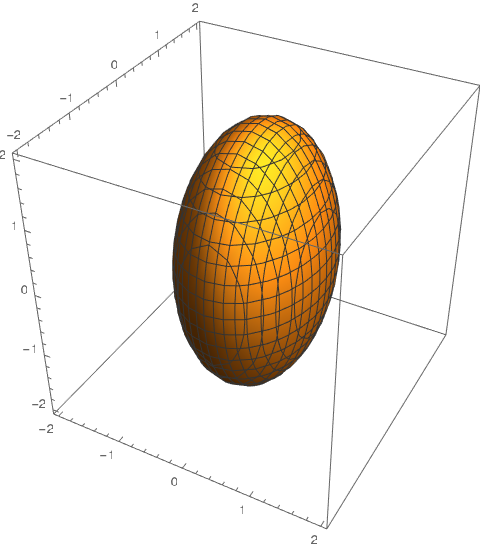#### Elliptic Paraboloid

• Horizontal traces (cross sections formed by intersection with xy plane) are ellipses
• Vertical traces are parabolas
• Axis of the parabola is the one that’s different (the one with degree one in the equation)
• $\frac{z}{c} = \frac{x^2}{a^2} + \frac{y^2}{b^2}$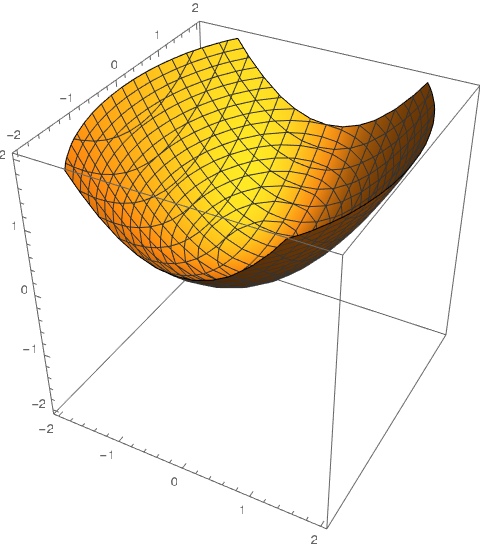#### Hyperbolic Paraboloid

• Horizontal traces (cross sections formed by intersection with planes parallel to the xy plane) are hyperbolas
• Vertical traces (cross sections formed by intersection with planes parallel to the yz and xz planes) are parabolas
• $\frac{z}{c} = \frac{x^2}{a^2} -\frac{y^2}{b^2}$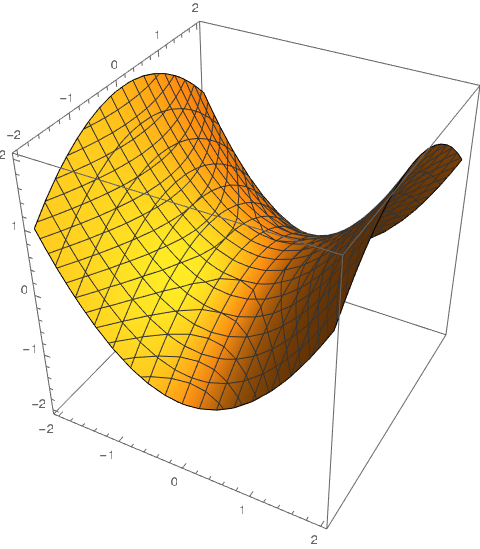#### Cone

• Horizontal traces are ellipses
• Vertical traces are planes (except when x = 0 and y = 0 when the traces are a pair of lines)
• $\frac{z^2}{c^2} = \frac{x^2}{a^2} + \frac{y^2}{b^2}$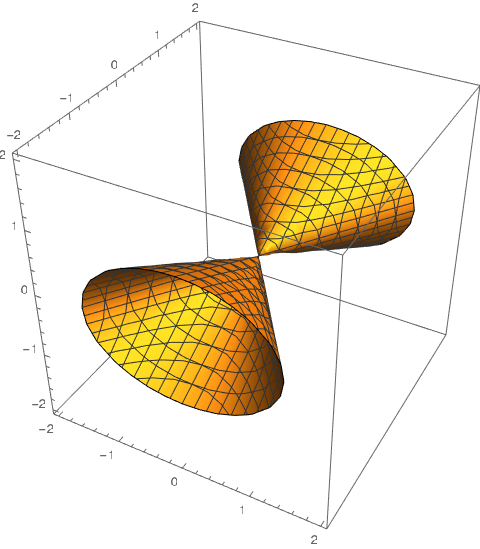#### Hyperboloid of One Sheets

• Horizontal traces are ellipses
• Vertical traces are hyperbolas
• $\frac{x^2}{a^2} + \frac{y^2}{b^2} - \frac{z^2}{c^2} = 1$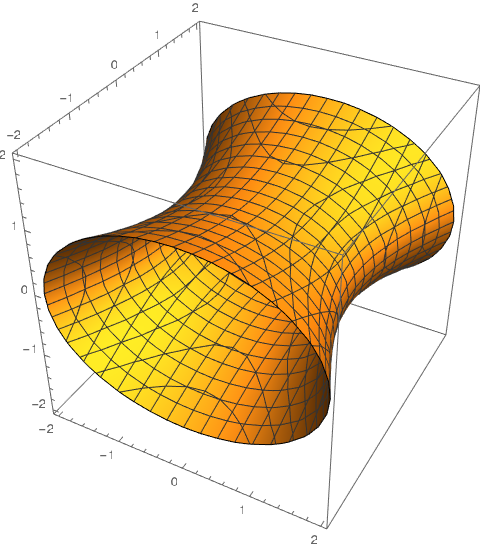#### Hyperboloid of Two Sheets

• Horizontal traces are ellipses if they exist
• Vertical traces are hyperbolas
• $-\frac{x^2}{a^2} - \frac{y^2}{b^2} + \frac{z^2}{c^2} = 1$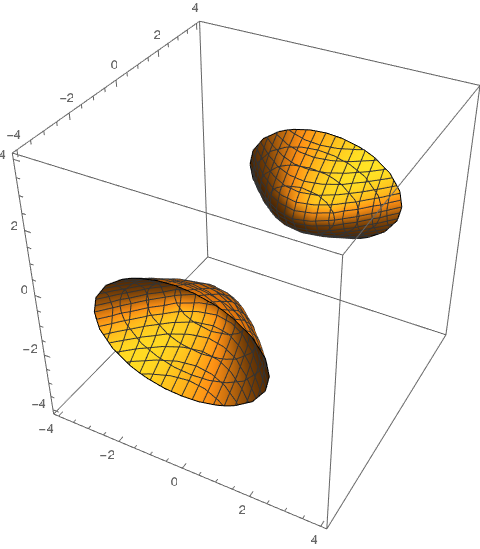## 13.1: Vector Functions and Space Curves

Vector Function: Domain: A set of real numbers, Range: A set of vectors

• Let $\vb{r}$ be a vector function for 3-d vectors
• The components of $\vb{r}$ are $f$, $g$, and $h$.
$$\vb{r}(t) = \ev{f(t), g(t), h(t)} = f(t)\vb{i} + g(t) \vb{j} + h(t) \vb{k}$$

### Limits and Continuity

$$\vb{r}(t) = \ev{f(t), g(t), h(t)}$$
$$\lim_{t \to a} \vb{r}(t) = \ev{\lim_{t \to a} f(t), \lim_{t \to a} g(t), \lim_{t \to a} h(t)}$$
As long as the limits of the component functions exist.

$\vb{r}$ is continuous at $a$ if $\lim_{t\to a} \vb{r}(t) = \vb{r}(a)$

### Space Curves

• $C$ is the set of all points $(x, y, z)$ where $x = f(t), y = g(t), z = h(t)$ (Parametric equations of C with $t$ as the parameter)
• Space Curve: The set of points in $C$
• $C$ is traced out by the tip of the vector $\vb{r}(t)$

### Drawing Curves

• To sketch parametric equations, remove $t$ from the equation and get the equations in terms of the $x$, $y$, and $z$ instead
• Then you can draw those equations on the x-y, y-z, and x-z planes. These “shadows” will represent the projections of the actual curve onto those planes.

## 13.2: Derivatives and Integrals of Vector Functions

$$\frac{d \vb{r}}{dt} = \vb{r}'(t) = \lim_{h \to 0} \frac{\vb{r}(t+h) - \vb{r}(t)}{h}$$
Unit Tangent Vector
$$\vb{T}(t) = \frac{\vb{r}'(t)}{|\vb{r}'(t)|}$$

If $f$, $g$, and $h$ are differentiable and $\vb{r}$ is a vector function
$$\vb{r}'(t) = \ev{f’(t), g’(t), h’(t)}$$

### Differentiation Rules

1. $\frac{d}{dt}[\vb{u}(t) + \vb{v}(t)]=\vb{u}'(t) + \vb{v}'(t)$
2. $\frac{d}{dt}[ c\vb{u}(t)] = c\vb{u}'(t)$
3. $\frac{d}{dt}[f(t)\vb{u}(t)] = f’(t) \vb{u}(t) + f(t) \vb{u}'(t)$
4. $\frac{d}{dt}[ \vb{u}(t) \cdot \vb{v}(t)] =\vb{u}'(t) \cdot \vb{t} + \vb{u}(t) \cdot \vb{v} ‘(t)$
5. $\frac{d}{dt} [\vb{u}(t) \cross \vb{v}(t)] = \vb{u}'(t) \cross \vb{v}(t) + \vb{u}(t) \cross \vb{v}'(t)$
6. $\frac{d}{dt}[ \vb{u}f(t)] = f’(t)\vb{u}'(f(t))$

### Integrals

$$\int_{a}^{b} \vb{r}(t) dt = \left(\int_{a}^{b} f(t)dt\right) \vb{i} + \left(\int_a^b g(t)dt \right) \vb{j} + \left(\int_a^b h(t)dt\right)\vb{k}$$

## 13.3: Arc Length and Curvature

### Length of a Curve

$$L = \int_a^b \sqrt{\left(\frac{dx}{dt}\right)^2 + \left(\frac{dy}{dt}\right)^2 + \left(\frac{dx}{dt}\right)^2} dt= \int_{a}^{b} |\vb{r}'(t)| dt$$

Note that a space curve can be represented by different bu equivalent vector functions (e.g. $r(t) = \ev{t, t^2, t^3}$ is equivalent to $r(u) = \ev{e^u, e^{2u}, e^{3u}}$).
These different representations are called parameterizations of the curve and the arc length calculation does not change with the choice of parameterization.

#### The Arc Length Function

The arc length, $s$, can be defined calculated using the following:
$$s(t) = \int_a^t \sqrt{\left(\frac{dx}{dt}\right)^2 + \left(\frac{dy}{dt}\right)^2 + \left(\frac{dx}{dt}\right)^2} dt$$

Sometimes a curve is parametrized with respect to arc length which means redefining $r(t)$ in terms of $s$ instead of $t$.

#### Curvature

A curve is smooth on an interval if $r'$ is continuous and $r’(t) \neq 0$ on an interval.
Tangent unit vector, $T$:
$$\vb{T}(t) = \frac{\vb{r}'(t)}{|\vb{r}'(t)|}$$

Curvature of a curve:
$$\kappa = \left| \frac{d\vb{T}}{ds} \right|$$
$$\kappa (t) = \frac{|\vb{T}'(t)|}{|\vb{r}'(t)|} = \frac{|\vb{r}'(t) \cross \vb{r}''(t)|}{|\vb{r}'(t)|^3}$$

### Normal and Binormal Vectors

• Unit Normal Vector: $\vb{N}(t)$ is a special vector that is orthogonal to $\vb{T}$ (indicates where curve is turning)
$$\vb{N}(t) = \frac{\vb{T}'(t)}{|\vb{T}'(t)|}$$
Note that $\vb{T}(t) \cdot \vb{T}'(t) = 0$, so the derivative of $\vb{T}$ is orthogonal to $\vb{T}$.

• Binormal Vector: Also a unit vector but orthogonal to both $\vb{T}$ and $\vb{N}$
$$\vb{B}(t) = \vb{T}(t) \cross \vb{N}(t)$$

• Normal Plane: Plane that contains $\vb{N}$ and $\vb{B}$. The plane contains all lines orthogonal to $\vb{T}$

• Osculating Plane: Plane that contains $\vb{N}$ and $\vb{T}$. The plane is the plane that comes closest to containing the part of the curve near the point of the tangent.

## 13.4: Motion in Space: Velocity and Acceleration

Velocity Vector v(t):
$$\vb{v}(t) = \lim_{h\to 0} \frac{\vb{r}(t+h) -\vb{r}(t)}{h} = \vb{r}'(t)$$

Speed:
$$|\vb{v}(t)| = |\vb{r}'(t)| = \frac{ds}{dt}$$

Acceleration:
$$\vb{a}(t) = \vb{v}'(t) = \vb{r}''(t)$$

### Projectile Motion

Assuming Gravitational acceleration of, $g$ and no air resistance

$$x = (v_0 \cos \alpha )t, \ \ \ \ y = (v_0 \sin \alpha ) t - \frac{1}{2} gt^2$$

### Tangential and Normal Components of Acceleration

$$\vb{a} = v’\vb{T} + \kappa v^2 \vb{N}$$
$$a_T = v’ = \frac{\vb{v} \cdot \vb{a}}{v} = \frac{\vb{r}'(t) \cdot \vb{r}''(t)}{|\vb{r}'(t)|}$$
$$a_N = \kappa v^2 = \frac{|\vb{r}'(t) \cross \vb{r}''(t)}{|\vb{r}'(t)|}$$

## 14.1: Functions of Several Variables

• Function of two variables: Assigns ordered pair $(x, y)$, in a set $D$ a unique real number. $D$ is the domain and the range is the set. The function is denoted by $z = f(x, y)$, where $x$ and $y$ are independent variables and $z$ is the dependent variable. For a function of two variables, the domain is a subset of $\mathbb{R}^2$ and the range is a subset of $\mathbb{R}$ (the range is always like this).

### Domain and Range

• A function can be visualized as a mapping between the domain and range, where each value in the domain only maps to one value in the range
• When graphing the domain, the number of dimensions is equal to the number of independent variables
• The range’s dimensions is always one

### Graphs

• Another way to visualize functions is by graphing

• The number of dimensions required to graph a function is the number of independent variables + the number of dependent variables

• This means the dimensions of the graph of the functions is always independent variables + 1
• For $z = f(x, y)$, the graph can thought of as a surface above or below the xy plane, where the domain is the xy plane

### Level Curves (Contour Plots)

• The third way to visualize functions is with contour plots also known as level curves
• For functions of two variables, the level curves are the curves with equations
$$f(x, y) = k$$
where $k$ is a constant in the range of $f$.
• Examples of contour plots are in topological maps that indicate how steep and high mountains or hills are
• When level curves are closer together, the surface is “steeper”
• For functions of two variables, the equation $f(x, y) = z = ax + by + c$ is called the linear function and represents a plane

## 14.3: Partial Derivatives

The $\textbf{partial derivative of } \mathbf{f} \textbf{ with respect to } \mathbf{x} \textbf{ at }\mathbf{(a, b)}$

$$f_x(a, b) = g’(a) \ \ \text{where } g(x) = f(x, b)$$

$$f_x(x, y) = \pdv{}{x} f(x, y) = \lim_{h\to 0} \frac{f(x+h, y) - f(x, y)}{h}$$
$$f_y(x, y) = \pdv{}{y} f(x, y) = \lim_{h\to 0} \frac{f(x, y + h) - f(x, y)}{h}$$

• To find the partial derivative with respect to $x$, hold $y$ constant
• To find the partial derivative with respect to $y$, hold $x$ constant

### Interpretations of Partial Derivatives

$$z= f(x, y)$$

• For the above function/surface, if we have a point $P(a, b, c)$, then when we hold $x$ constant with $x = a$, we get a curve, since the plane $x = a$ intersected with the surface, $f$
• $g’(a) = f_x(a, b)$ represents the line tangent to the curve at that point.

### Higher Derivatives

$$(f_{x})_{y} = f_{xy} = \pdv{}{y}\left(\pdv{f}{x}\right) = \pdv{f}{y}{x}$$

Clairaut’s Theorem:
If $F$ is defined on a disk, $D$, that contains point $(a, b)$, and both $f_{xy}$ and $f_{yx}$ are continuous on the disk, then $f_{xy}(a, b) = f_{yx}(a, b)$

### Partial Differential Equations

• Partial Differential Equations: Used to express physical laws and describe phenomina

• Laplace’s equation: The following partial differential equation (2 dimensional version):
$$\pdv{u}{x} + \pdv{u}{y} = 0$$

• Harmonic Functions: The solutions to Laplace’s equation

• Used in heat conduction, fluid flow, electric potential, etc
• Wave Equation: Describes waves
$$\pdv{u}{t} = a^2 \pdv{u}{x}$$

## 14.4: Tangent Planes and Linear Approximation

### Tangent Planes

If $f$ has continuous partial derivatives, the equation of the tangent plane at a point is the following:
$$z - z_0 = f_x(x_0, y_0) (x - x_0) + f_y(x_0, y_0)(y-y_0)$$

This is similar to how in single-variable calculus, the equation of the tangent line was the following:
$$y-y_0 = f’(x_0)(x - x_0)$$

### Linear Approximations

You can use the tangent plane at a point to approximate a function at a nearby point like in single-variable calculus.

## 14.5: Chain Rule

From single variable calculus
$$\frac{df}{dx}= \frac{d}{dx}\left(f(g(x))\right) = f’(g(x)) g’(x) = \frac{df}{dg}\frac{dg}{dx}$$

For multivariable:
$$\frac{dw}{dt} = \frac{d}{dt} \left(x(t), y(t)\right) = \pdv{w}{x} \dv{x}{t} + \pdv{w}{y} \dv{y}{t}$$

## 14.6: Directional Derivative and Gradient

### Directional Derivative

If $f$ is differentiable, $f$ has a directional derivative in the direction of the unit vector $\vb{u} = \ev{a, b}$
$$D_u f(x, y) = f_x(x, y)a + f_y(x, y)b = \ev{f_x(x, y), f_y(x, y)} \cdot \vb{u}$$

$$\nabla f = \text{del } f = \ev{f_x(x, y), f_y(x, y)}$$

### Maximizing the Directional Derivative

• Q. If we have a function $f$, and consider all directional derivatives at a point, then which directional derivative is greatest (“steepest” or has the fastest rate of change)
• A. When $\vb{u}$ is in the same direction as the gradient vector since that will maximize the directional derivative

### Equation of Tangent Plane to Level Surface

$$\pdv{F}{x} \dv{x}{t} + \pdv{F}{y} \dv{y}{t} + \pdv{F}{z} \dv{z}{t} = 0$$
$$\nabla F \cdot \vb{r}'(t) = 0$$
$$F_x(x_0, y_0, z_0) (x-x_0) + F_y(x_0, y_0, z_0) (y - y_0) + F_z(x_0, y_0, z_0)(z-z_0)=0$$

## 14.7: Maximums and Minimums (Optimization)

$z=f(x, y)$ to be optimized

Local max at $(x_0, y_0)$ if $f(x, y) \le f(x_0, y_0)$ for all points $(x, y)$ near $(x_0, y_0)$

### 1st Derivative Test

Local extrema at $(a, b)$ if $\nabla f(a, b) = DNE$ or $\nabla f(a, b) = 0$ (Converse is not true)

### 2nd Derivative Test

Suppose $\nabla f(a, b) = \ev{0, 0}$

Calculate the following
$$D = det\left(\begin{bmatrix} f_{xx} & f_{xy} \\ f_{yx} & f_{yy} \end{bmatrix} \right) = f_{xx} f_{yy} - f_{xy}^2$$

1. If $D(a, b) < 0$, there is no local extrema, but there is a saddlepoint
2. If $D(a, b) = 0$, no conclusion is possible
3. If $D(a, b) > 0$, then calculate $f_{xx}$
• If $f_x(a, b) > 0$, there is a local min
• If $f_x(a, b) < 0$, there is a local max
• If $f_x(a, b) = 0$, no conclusion is possible

## 15.1: Double Integrals over Rectangles

Volume $V$ of a solid above the rectangle $R$ and below the surface $z = f(x, y)$:
$$V = \iint\limits_R f(x, y) dA$$
where $dA$ represents the area of the sub-rectangles that compose the large rectangle $R$.

### Fubini’s Theorem

If $f$ is continuous on the rectangle $R = \{(x, y) | a \le x \le b, c\le y \le d \}$, then
$$\iint\limits_R f(x, y) dA = \int_a^b \int_c^d f(x, y) \, dy \, dx = \int_d^c \int_a^b f(x, y) \, dx \, dy$$

If $R = [a, b] \cross [c, d]$
$$\iint\limits_R g(x) \, h(y) \, dA = \int_a^b g(x) \, dx \int_c^d h(y) \, dy$$

## 15.2: Double Integrals over General Regions

• We can take the double integral over a general region $D$ instead of just a rectangle
• Let $F(x, y)$ be defined as the following:
$$F(x, y) = \begin{cases} f(x, y) & \text{if }(x, y) \text{ is in } D \\ 0 & \text{if }(x, y) \text{ is in } R \text{ but not in } D \end{cases}$$

$$\iint\limits_{D} f(x, y) dA = \iint\limits_R F(x, y) dA$$

• If $D$ is lies between two continuous functions, $g_1(x)$, and $g_2(x)$ and if $f$ is continuous on a region $D$ such that
$$D = \{ a \le x \le b, g_1(x) \le y \le g_2(x)\}$$
then
$$\iint\limits_{D} f(x, y)\, dA = \int_a^b \int_{g_1(x)}^{g_2(x)} f(x, y) \, dy \, dx$$

• If $f$ is continuous on a region $D$ such that
$$D = \{ c \le y \le d, h_1(y) \le y \le h_2(y)\}$$
then
$$\iint\limits_{D} f(x, y)\, dA = \int_b^c \int_{h_1(y)}^{h_2(y)} f(x, y) \, dx \, dy$$

### Properties of Double Integrals

1. $$\iint\limits_D \left(f(x, y) + g(x, y)\right) \, dA = \iint\limits_D f(x, y) \, dA + \iint_D g(x, y) \, dA$$
2. If $c$ is a constant, $$\iint\limits_D cf(x, y) \, dA = c \iint\limits_D f(x, y) \, dA$$
3. If $f(x, y) \ge g(x, y)$ for all $x, y$ in $D$
$$\iint\limits_D f(x, y) \, dA \ge \iint\limits_D g(x, y) \, dA$$
4. If $D = D_1 \cup D_2$, and $D_1$ and $D_2$ don’t overlap
$$\iint\limits_D f(x, y)\, dA = \iint\limits_{D_1} f(x, y) \, dA + \iint\limits_{D_2} f(x, y) \, dA$$
5. $$\iint\limits_D 1 \, dA = A(D) = \text{Area of }D$$
6. If $m \le f(x, y) \le M \; \forall (x, y) \in D$
$$m \, A(D) \le \iint\limits_D f(x, y) \, dA \le M\,A(D)$$

## 15.3: Double Integrals in Polar Coordinates

$$x = r \cos \theta \;\;\;\;\; y = r \sin \theta \;\;\;\;\; x^2 + y^2 = r^2$$

### Double Integrals over Polar Rectangles

$$\iint\limits_R f(x, y) \, dA = \int_{\alpha}^{\beta} \int_b^a f(r \cos \theta, \, r \sin \theta) r \, dr \,d\theta$$

### Double Integrals over General Polar Regions

$$\iint\limits_D f(x, y) \, dA = \int_{\alpha}^{\beta} \int_{h_1(\theta)}^{h_2(\theta)} f(r \cos \theta, \, r \sin \theta) r \, dr \,d\theta$$

## 15.5: Surface Area

For a function $f(x, y)$, the surface area can be computed with the following:
$$A(S) = \iint\limits_D \sqrt{f_x(x, y)^2 + f_y(x, y)^2 + 1} \; dA$$

## 15.7: Triple Integrals with Cylindrical Coordinates

Cylindrical to rectangular
$$x = r \cos \theta, \ \ \ y = r \sin \theta, \ \ \ z = z$$

Rectangular to Cylindrical
$$r^2 = x^2 + y^2, \ \ \ \tan \theta = \frac{y}{x}, \ \ \ z = z$$

## 15.8: Triple Integrals with Spherical Coordinates

$$x = \rho \sin \phi \cos \theta, \ \ \ y = \rho \sin \phi \sin \theta, \ \ \ z= \rho \cos \phi$$

$$\rho^2 = x^2 + y^2 + z^2$$

$$\iiint\limits_{E} f(x, y, z) \ dV= \int_c^d \int_\alpha^\beta \int_a^b f(\rho \sin \phi \cos \theta, \rho \sin \phi \sin \theta, \rho \cos \phi) \ \rho^2 \sin \phi \ d \rho \ d\phi \ d\theta$$

## 16.2: Line Integrals

### Line Integrals of Functions

$C$ is a curve

$$\int_{C} f(x, y) \ \ ds= \int_{a}^{b} f(x(t), y(t)) \ \ \sqrt{\left(\frac{dx}{dt}\right)^2 + \left(\frac{dy}{dt}\right)^2} \ \ dt$$

### Line integrals of Vector Fields

Calculate a line integral along a curve $C$ in a vector field $\vec{F}$

$$\int\limits_C \vec{F} \cdot d\vec{r} = \int\limits_C \vec{F} \cdot \vec{T} \ ds$$
where $\vec{T}$ is a unit tangent vector to $C$ in the direction of increasing $T$

Calculation (in general): use parametrization
$$= \int_a^b \vec{F}\left( \vec{r}(t) \right) \cdot \vec{r}’ (t) \ dt$$

## 16.7: Surface Integrals (of Functions)

$S$ is a surface given by $z = f(x, y)$ where $(x, y) \in D$

$$\iint\limits_S g(x, y, z) \ dS = \iint\limits_D g(x, y, f(x, y)) \sqrt{1 + f_x(x, y)^2 + f_y(x,y)^2} \ dA$$

For surface area, $g(x, y, z) = 1$

## 16.4: Green’s Theorem

To evaluate a line integral:
$$\int_C \vec{F} \cdot d\vec{r}$$
Three options

1. From definition: use a parametrization $\vec{r}(t)$ for C, etc.
2. Use Fundamental Theorem: only works if $\vec{F}$ is conservative and needs a potential
3. Green’s Theorem: Needs C to be closed

### Given

• A curve $C$ (“piecewise smooth”) in $xy$-plane
• closed: start = end
• simple: does not cross itself (no figure 8)
• positively oriented (counterclockwise) - easy adjustment if clockwise
• $D$ (region in the $xy$-plane
• a vector field $\vec{F} = \ev{P(x, y), Q(x, y)}$

### Green’s Theorem

$$\int_C \vec{F} \cdot d\vec{r} = \iint\limits_{D} (Q_x - P_y) \ \ dA$$

Remarks

1. Green’s Theorem implies the criterion (earlier)
$$\vec{F} \text{ conservative } \iff P_y = Q_x$$
• Forward implication follows from Clairaut’s Theorem
• Reverse implication follows from Green’s Theorem
1. Right Hand Side (RHS) is usually earlier than the LHS

### Example

Evaluate $\oint x^2 y^2 \ dx + xy \ dy$

$C$: arc of the parabola $y=x^2$ from $(0,0)$ to $(1, 1)$ followed by the line segments $(1, 1)$ to $(0, 1)$ and from $(0,1)$ to $(0,0)$

$$P = x^2 y^2, \ \ Q = xy$$

$$P_y = 2yx^2, \ \ Q_x = y$$

$$\int_C \vec{F} \cdot d\vec{r} = \iint\limits_D (y - 2yx^2) \ dA$$

$$= \int_{0}^{1} \int_{x^2}^{1} (y - 2yx^2)\ dy \ dx$$
$$= \int_0^1 \left. \frac{y^2}{2} - y^2 x^2 \right\vert_{y = x^2}^{y = 1} dx = \int_0^1 \left(-\frac{x^4}{2}+ x^4 + (\frac{1}{2} - x^2)\right) dx$$

### Example

$$\oint \vec{F} \cdot d\vec{r}$$
$$\vec{F} = \ev{e^{-x} + y^2 , e^{-y} + x^2}$$
$C$: Arc of the curve $y = \cos x$ from $(-\frac{\pi}{2}, 0)$ to $(\frac{\pi}{2} , 0)$ followed by the line segment back from $(\frac{\pi}{2}, 0)$ to $(\frac{-\pi}{2}, 0)$

Clockwise!

Rule: For any curve $C$, $-C$ denotes $C$ with the direction reversed

### Outlook

Green’s Theorem implies the earlier criterion: $P_y = Q_x \implies \vec{F} \text{ is conservative }$
$$\int_C \vec{F} \cdot d\vec{r} = \iint_D \underbrace{0}_{Q_x - P_y = 0} dA = 0$$

for any closed, single curve (any orientation), $C$

It then follows that, for any curve $C$ (not necessarily closed), $\int_C \vec{F} \cdot d\vec{r}$ only depends on the end points of $C$

• Independence of path

## 16.4: Curl and Divergence (n = 3)

3-dimensions

Given: $\vec{F} = \ev{P(x, y, z), Q(x, y, z), R(x, y, z)}$

Let $\nabla = \ev{\pdv{}{x}, \pdv{y}, \pdv{t}}$

then for a function $f = f(x, y, z)$
$\nabla f = \ev{\pdv{x} f, \pdv{y} f, \pdv{z} f}$ as we know already (gradient)

### Definition

$$div \vec{F} = \nabla \cdot \vec{F} = \pdv{x} P + \pdv{y} Q + \pdv{z} R =P_x + Q_y + R_z$$

$$curl \vec{F} = \nabla \cross \vec{F} = \begin{bmatrix} \hat{i} & \hat{j} & \hat{k} \\ \pdv{x} & \pdv{y} & \pdv{z} \\ P & Q & R \end{bmatrix} = \ev{R_y - Q_z, P_z - R_x, Q_x - P_y}$$

### Theorem

$$\vec{F} \text{ conservative } \iff \curl\vec{F} = \ev{0, 0, 0}$$

Remarks

1. Generalizes our old criterion for conservativity of $\vec{F} = \ev{P(x, y), Q(x, y), 0}$
2. Again (as for n=2), the implication $\implies$ follows from Clairaut’s Theorem

$$\vec{F} \text{ is conservative} \implies \exists f: \nabla f = \vec{F} \implies \curl \vec{F} = \nabla \cross \nabla f = \ev{0, 0, 0}$$

#### Example

$$\vec{F} = \ev{xyz^4, x^2 z^4, 4x^2 yz^3}$$

• Is the above conservative?
• If so, find a potential

$$\nabla \cross \vec{F} = \ev{R_y - Q_z, P_z - R_x, Q_x - P_y}$$

$$P_y = xz^4$$
$$Q_x = 2xz^4$$

$xz^4 \neq 2xz^4$, so $\vec{F}$ is not conservative

### Facts

1. $\vec{F}$ is conservative $\iff$ curl $\vec{F} = \vec{0}$
2. div(curl($\vec{F}$)) = 0

## 16.7: Surface Integrals (Of Vector Fields)

### New: Surface Integrals of Vector Fields

• Only for surfaces of the form
$$S: z = g(x, y) \ \ \ \ (x, y) \in D$$

$$\iint\limits_S \vec{F} \cdot d\vec{S} = \iint\limits_S \vec{F} \cdot \underbrace{\vec{n}}_{\text{unit normal vector}} \ dS$$

$$= \iint\limits_D \vec{F} \cdot \vec{n} \sqrt{1 + g_x^2 + g_y^2} \ dA$$

#### Applications

“Flux” of $\vec{F}$ across the surface $S$

• $> 0$ means more gas flows through $S$ in the direction of $\vec{n}$ (upwards) than the opposite direction

#### In detail

• Upward orientation
$$\vec{n} = \frac{\ev{1, 0, g_x} \cross \ev{0, 1, g_y}}{|\ev{1, 0, g_x} \cross \ev{0, 1, g_y}|} = \frac{\ev{-g_x, -g_y, 1}}{\sqrt{1+g_x^2 + g_y^2}}$$

where $\ev{1, 0, g_x}$ and $\ev{0, 1, g_y}$ are tangent vectors to a point on $S$

#### Formula (For calculations)

Assuming $n$ is upwards (if downwards, then negate the formula for $n$)
$$\vec{F} = \ev{P, Q, R}$$
$$\vec{n} = \frac{\ev{-g_x, -g_y, 1}}{\sqrt{1+g_x^2 + g_y^2}}$$

$$\iint\limits_{S} \vec{F} \cdot d\vec{S} = \iint\limits_D \vec{F} \cdot \vec{n} \sqrt{1 + g_x^2 + g_y^2} \ dA$$
$$\iint\limits_{S} \vec{F} \cdot d\vec{S} = \iint\limits_D \vec{F} \cdot \ev{-g_x, -g_y, 1} \ dA= \iint\limits_D \left(-P \ g_{x} - Q \ g_{y} + R\right) \ dA$$

#### Example

$$\vec{F} = \ev{-x, -y, z^3}$$

• $S$: part of the cone $z = \sqrt{x^2 + y^2}$
between the surfaces $z = 1$ and $z = 3$
• Orientation: Downwards

$$g_x = \frac{x}{x^2 + y^2}$$
$$g_y = \frac{y}{x^2 + y^2}$$

$$\iint\limits_{S} \vec{F} \cdot d\vec{S} = - \iint\limits_D (-P g_x - Q g_y + R) \ dA$$
$$= - \iint\limits_{D} \left(\frac{x^2}{\sqrt{x^2 + y^2}} + \frac{y^2}{\sqrt{x^2 + y^2}}\right) + (z^3) \ dA$$
$$= -\iint\limits_{D} \left(\frac{x^2}{\sqrt{x^2 + y^2}} + \frac{y^2}{\sqrt{x^2 + y^2}}\right) + (x^2 + y^2)^{3/2} \ dA$$
$$= - \int_{0}^{2\pi} \int_{1}^{3} (r^2 + r^4) \ dr \ d\theta = - \frac{1712}{15} \pi$$

Application: Net flow of gas is up since flux is negative and normal is down

## 16.8: Stokes’ Theorem

Calculates result for surface integral in a certain case

$$\iint\limits_S (curl \ \vec{F}) \cdot d\vec{S} = \oint\limits_C \vec{F} \cdot d\vec{r}$$

• $S$ is an oriented (up or down) surface in $\mathbb{R}^3$
• $\vec{F} = \ev{P, Q, R}$
• $C$: Closed curve that forms boundary of the surface $S$ in $\mathbb{R}^3$ oriented according to Right Hand Rule (RHR)

### Special Case of Stokes’ Theorem

$$\vec{F} = \ev{P, Q, 0}$$
$$P= P(x, y) \ \ \ Q = Q(x, y)$$

$$curl \ \vec{F} = \ev{0, 0, Q_x - P_y}$$

$$(x, y) \in D$$

$$S = D \text{ in the xy plane} = \text{interior of curve }C$$

Stokes’ Theorem states:
$$\oint\limits_C \vec{F} \cdot d\vec{r} = \iint\limits_D (Q_x - P_y) \ dA$$

• Note that the above result is just Green’s Theorem
• Stokes’ Theorem can be viewed as a general version of Green’s Theorem

### Example

Calculate the following
$$\iint\limits_S \left(curl \ \vec{F}\right) \cdot d \vec{S}$$

$$\vec{F} = \ev{x^2 z^2 , yz^2, \tan^{-1} (x^2 yz^2)}$$
$$S: z = \sqrt{x^2+y^2} \text{ cone oriented up} \ 0 \le z \le 2$$

Boundary curve $C$ (counter-clockwise by RHR)
$$x^2 + y^2 = 4$$

Parametrization is still counter-clockwise
$$C: \vec{r}(t) = \ev{ 2\cos t,2 \sin t, 2}$$

$$\iint\limits_S (curl \ \vec{F})\cdot d\vec{S} = \oint\limits_C \vec{F} \cdot d\vec{r}$$
Just calculate line integral

## 16.9 Divergence Theorem (Dim 3)

Given

• $\vec{F} = \ev{P, Q, R}$
• $E$ as a solid in $\mathbb{R}^3$ (“simple” $\implies$ bounded)
• $S = \partial E$ (boundary of $E$ oriented upwards)

$$\iint\limits_{S} \vec{F} \cdot \vec{dS} = \iiint\limits_{E} div\ \vec{F} \ dV$$

### Example

$$\vec{F} = \ev{x^2 y z , xy^2 z, xyz^2}$$

S = the box enclosed by the following
$$x= 0, x = a, y = 0, y=b, z = 0. z =c$$

Orientation is outwards

$$\iint\limits_{S} \vec{F} \cdot \vec{dS} = \iiint\limits_{E} div \ \vec{F} \ dV$$
$$= \int_0^a \int_0^b \int_0^c div \ \vec{F} \ dz \ dy \ dx$$

$$= \int_0^a \int_0^b \int_0^c div \ \vec{F} \ dz \ dy \ dx = a^2b^2c^2 \div F$$

### Example

$$\vec{F} = \ev{z, y, zx}$$
S = surface of the tetrahedron enclosed by the coordinate planes & the plane
$$\frac{x}{a} + \frac{y}{b} + \frac{z}{c} = 1$$

E = inside tetrahedron

$$\iint\limits_{S} \vec{F} \cdot \vec{dS} = \iiint\limits_{E} div\ \vec{F} \ dV$$
$$\iiint\limits_{E} (1 +x) \ dV$$
$$0 \le x \le b$$
$$0 \le y \le b\left(1 - \frac{x}{a}\right)$$
$$0\le z \le c\left(1 - \frac{x}{a} - \frac{y}{b}\right)$$

$$\int_0^{a} \int_{0}^{b(1-\frac{x}{a})} \int_0^{c(1-\frac{x}{a} - \frac{y}{b})} (1+x) dz \ dy \ dx$$

## Summary of Green’s, Stokes’, Divergence Theorems

1. Green’s Theorem
$$\vec{F} = \ev{P, Q}, \ \ \ P(x, y) = P, \ \ \ Q(x, y) = Q$$
$C$ closed curve in $xy$ plane ($\mathbb{R}$) oriented counter clockwise (positive orientation)
$$\oint\limits_C = \vec{F} \cdot d\vec{r} = \iint\limits_D (Q_x - P_y) \ dA$$
where $D$=Interior of $C$

2. Stokes’ Theorem
$$\vec{F} = \ev{P, Q, R}, \ \ \ P(x, y) = P, \ \ \ Q(x, y) = Q, \ \ \ R = R(x, y)$$
Closed curve $C$ in $\mathbb{R}^3$ oriented counter-clockwise when viewed from above

$$\oint\limits_C \vec{F} \cdot d\vec{r} = \iint\limits_S \curl F \cdot d\vec{s}$$ where $S$ is a surface in $\mathbb{R}$ with boundary $C$ and $\vec{n}$ oriented up

1. Divergence Theorem
$F$ is the same as in Stokes’
$S$ is a surface, closed in $\mathbb{R}^3$ oriented out
$$\iint \vec{F} \cdot d\vec{S} = \iiint\limits_{E} \div F \ \ dV$$
$E$ is a solid in the interior of $S$